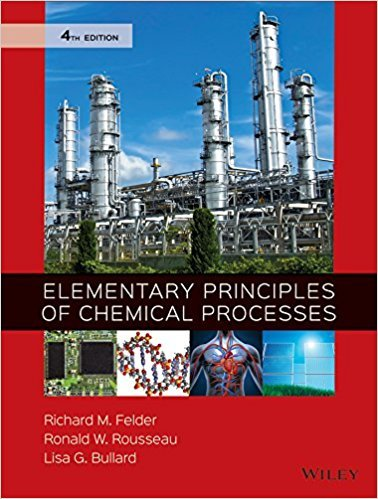×
×

# Solutions for Chapter 3.4c: PRESSURE## Full solutions for Elementary Principles of Chemical Processes | 4th Edition

ISBN: 9780470616291Solutions for Chapter 3.4c: PRESSURE

Solutions for Chapter 3.4c
4 5 0 266 Reviews
30
3
##### ISBN: 9780470616291

Since 4 problems in chapter 3.4c: PRESSURE have been answered, more than 38526 students have viewed full step-by-step solutions from this chapter. This textbook survival guide was created for the textbook: Elementary Principles of Chemical Processes, edition: 4. Chapter 3.4c: PRESSURE includes 4 full step-by-step solutions. Elementary Principles of Chemical Processes was written by and is associated to the ISBN: 9780470616291. This expansive textbook survival guide covers the following chapters and their solutions.

Key Chemistry Terms and definitions covered in this textbook
• activity

The decay rate of a radioactive material, generally expressed as the number of disintegrations per unit time. (Section 21.4)

• Alkyne

An unsaturated hydrocarbon that contains one or more carbon-carbon triple bonds.

• Allylic carbon

A carbon adjacent to a carbon-carbon double bond.

• alpha (a) position

The position immediately adjacent to a functional group.

One-half the distance between the two nuclei in two adjacent atoms of the same element in a metal. For elements that exist as diatomic units, the atomic radius is one-half the distance between the nuclei of the two atoms in a particular molecule. (8.3)

• carboxylic acid

A compound that contains the ¬COOH functional group. (Sections 16.10 and 24.4)

• electron density

The probability of finding an electron at any particular point in an atom; this probability is equal to c2, the square of the wave function. Also called the probability density. (Section 6.5)

• graft copolymer

A polymer that contains sections of one homopolymer that have been grafted onto a chain of the other homopolymer.

• inversion of configuration

During a reaction, when the configuration of a chirality center is changed.

• law of definite proportions

A law that states that the elemental composition of a pure substance is always the same, regardless of its source; also called the law of constant composition. (Section 1.2)

• petroleum

A naturally occurring combustible liquid composed of hundreds of hydrocarbons and other organic compounds. (Section 5.8)

• Reductive elimination

Elimination of two substituents at a metal center, causing the oxidation state of the metal to decrease by two.

• replacement test

A test for determining the relationship between two protons. The compound is drawn two times, each time replacing one of the protons with deuterium. If the two compounds are identical, the protons are homotopic. If the two compounds are enantiomers, the protons are enantiotopic. If the two compounds are diastereomers, the protons are diastereotopic.

• saponification

Hydrolysis of an ester in the presence of a base. (Section 24.4)

• significant figures

The digits that indicate the precision with which a measurement is made; all digits of a measured quantity are significant, including the last digit, which is uncertain. (Section 1.5)

• solution alloy

A homogeneous alloy, where two or more elements are distributed randomly and uniformly throughout the solid. (Section 12.3)

• spontaneous

A reaction with a negative DG, which means that products are favored at equilibrium.

• tosylate

An excellent leaving group (OTs). transition state (Sect. 6.6): A state through which a reaction passes. On an energy diagram, a transition state corresponds with a local maximum.

• Unimolecular reaction

A reaction in which only one species is involved in the rate-determining step

• Unsaturated hydrocarbon

A hydrocarbon containing one or more carbon-carbon double or triple bonds. The three classes of unsaturated hydrocarbons are alkenes, alkynes, and arenes

×